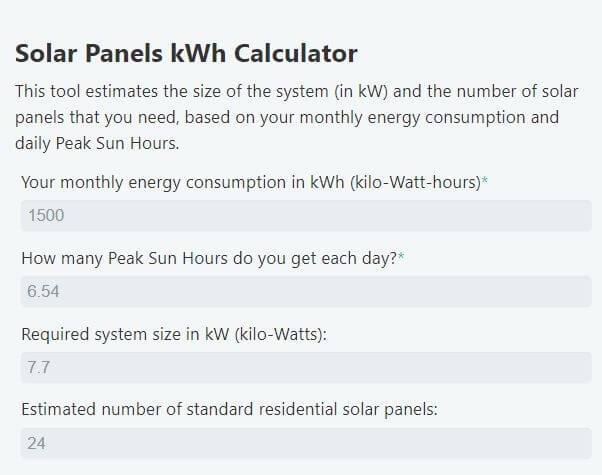# How many solar panels do I need for 1500 kWh per month?

The following article explains an easy way to estimate the size of the system in kW (kilo-Watts), and the number of solar panels that you need to offset 1500 kWh (kilo-Watt-hours) of monthly energy consumption.

## How many solar panels do I need for 1500 kWh per month

1500 kWh per month is equivalent to about 50 kWh of energy consumption per day. So, how many solar panels do you need to produce 50 kWh of energy per day?

On average, a solar energy system that produces 1500 kWh per month (50 kWh per day), would be rated at 10 kW. This is roughly equivalent to 30 residential solar panels. However, the size of a PV system that produces this much energy, will mainly depend on the amount of available sunlight.

For example, on average, a person in Iowa City, IA would need a 10.6 kW system consisting of about 32 residential solar panels to produce 1500 kWh per month. A person in Los Angeles, CA would only need an 8.2 kW system consisting of about 24 solar panels to produce the same amount of energy.

This is because the amount of direct sunlight that a solar panel receives differs from location to location. However, thankfully, the amount of sunlight that an area receives is measurable and somewhat predictable.

The energy that an area receives from the sun is measured in kWh/m² (kilo-Watt-hours per square meter) and is referred to as Peak Sun Hours. 1 Peak Sun Hour is equivalent to 1 kWh/m².

Peak Sun Hours are used to predict the amount of energy that a solar installation would produce. If we know how much energy needs to be produced, Peak Sun Hours can be used to determine the size of the system that we need (in kW).

System Wattage (kW) = Daily Energy production (kWh) ÷ Daily Peak Sun Hours

For example, according to the National Renewable Energy Laboratory (NREL), the city of Houston, TX receives – on average – 5.33kWh/m² of sunlight energy per day. This area can be said to receive 5.33 Peak Sun Hours per day.

To produce 1500 kWh of energy per month, the size of the system that a person in this location would need can be calculated as such:

System Wattage = Daily Energy production ÷ Daily Peak Sun Hours

System Wattage = 50 kWh ÷ 5.33

System Wattage = 9.38 kW

Therefore, a person in Houston, TX would need 9.38 kilo-Watts of solar power to produce 1500 kWh of energy per month. Such a system would – on average – consist of about 28 residential solar panels.

So, how many Peak Sun Hours do you get?

NREL’s PVWatts Calculator allows you to determine the average amount of sunlight (Peak Sun Hours) that you receive each day.After submitting your address, the tool estimates the number of peak sun hours for that area. For example, I submitted Tuscon, Arizona as my location. The results are shown in the image below:The PVWatts Calculator estimates that Tuscon, AZ gets 6.54 Peak Sun Hours per day. By using the formula above, I calculated that a person in this area would need 7.6 kW of solar power to produce 1500 kWh per month.

As soon as you’ve determined your Peak Sun Hour, you can use the following calculator to estimate the size of the system and the number of solar panels that you need.

## Solar panels kWh calculator

I used the peak sun hour from the previous example, and these are the results:In this example, the calculator estimates that the system would have to be rated at 7.7 kW or more to produce 1500 kWh per month. Such a system would consist of about 24 residential solar panels.

Please note that the Peak Sun Hours used in this example is an annual average. In the winter, the same location would receive a fewer amount of sunlight and therefore fewer Peak Sun Hours. In the summer, the same location would receive more than 6.54 peak sun hours.

However, the majority of home solar installations are grid-tied. This means that any excess energy produced by the solar panels will be stored in the grid, and used later when it needed.